# Python Tutorial: Python Decimal Module – Python Numbers #27

Read more about the Python Decimal Module at http://learnpythontutorial.com/python-decimal-module-python-tutorial/

Python Decimal Module
In the previous two Python tutorials, we looked at issues that arise when working collaboratively with floating point numbers in the Python programming language. When working with floating point numbers we have seen that Python sometimes returns a value that we are not suspecting. This is doesn’t happen often but when we get a number that is a few ten…

## 8 thoughts on “Python Tutorial: Python Decimal Module – Python Numbers #27”

1.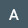Abhideep Singh says:

hi, are there any inbuilt functions in Python to round the number to the integer in the negative direction. Such as, fix in Matlab. eg fix(7.6) = 7

2.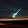Shubham Bhattacharya says:

well in case of importing decimal what's the difference between decimal and Decimal…what we do in decimal.Decimal(8.7) what's the difference?

3.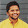Likes Technology says:

Do we have any way, to set the precision as per required, through out the programme …….bcos setting the PRECISION with GETCONTEXT is not at all a good idea and calling the QUANTIZE every time is not simple.. …………AND I WOULD LIKE TO THANK YOU FOR YOUR VALUABLE TUTORIALS …THOUGH IAM FROM A REMOTE AREA YOUR TUTORIAL MADE THE JoB EASY

4.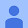Dylan Johnson says:

if we want to use decimals instead of int what would we type

5.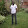Nguiya Passi Neo says:

Please how to use decimal in a loop for exemple to evaluate matrice multiplication and obtain a matrice with elements which contain 30 digit after the coma.

6.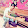Subramani Arulmani says:

Why don't I use round() instead of quantizing?

7.Shawn Brown says:

One of the primary benefits of using the decimal module is the ability to avoid many floating point problems from the start. But using Decimal(7.6) creates a floating point approximation of 7.6 and then passes this approximation into the Decimal constructor–the precision is immediately lost. The example would benefit from using Decimal("7.6"), etc.–this creates a true 7.6 value that can be operated on without fudging the result by lowering the decimal precision of the context:

>>> from decimal import Decimal
>>> Decimal('7.6') + Decimal('8.7')
Decimal('16.3')

There are no rounding issues to correct when you use precise values (the strings representations) to instantiate the Decimal objects. Yes, it's nice that the module gives us the tools to manage rounding issues but the ability to avoid them in the first place is the decimal module's real benefit.

8.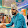Rohan Ghige says:

I found out that,
round(2.675,2) gives 2.67; but
round(2.6751,2) gives 2.68, and also round(2.6756,2) gives 2.68.

What is reason behind this?